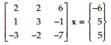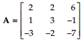Create an Account

Home / Questions / Crout’s method uses Crout factorization see Problem 57 of the coefficient matrix A of the ...

Crout’s method uses Crout factorization see Problem 57 of the coefficient matrix A of the linear system Ax b and generates two triangular systems which can be solved by back and forward

Crout’s method uses Crout factorization (see Problem 57) of the coefficient matrix A of the linear system Ax = b and generates two triangular systems, which can be solved by back and forward substitution. Write a user-defined function with function call x = Crout_ Method(A,b). Apply Crout_Method toProblem 57

Crout LU factorization requires the diagonal entries of U be 1’s, while L is a general lower-triangular matrix. Perform direct calculation of the entries of L and U for the case of a 3 × 3 matrix, similar to that in Example 4.6. Based on the findings, write a user-defined function with function call [L U] = Crout_Factor(A) that returns the desired lower- and upper-triangular matrices for any n × n matrix. Apply Crout_Factor toJul 22 2020 View more View LessSubscribe To Get Solution